November 16, 2021

# Class 10 Maths Chapter 1 Real Numbers – Complete Study Material

In CBSE class 10 maths chapter 1 Real numbers, we will study real numbers in-depth. The base for this chapter has already been set in class 9th where we came to know about rational and irrational numbers. Also, many other topics that we will learn in detail herein class Xth has already been introduced to you in the previous class. So, if you remember what you had studied in class IXth about real numbers, then things will get a lot easier for you.

## Class 10 Maths Chapter 1 Real Numbers

Here are some of the important terms from the previous class  that you should  know before initiating the preparation for this chapter:

What are real numbers?

In simple words, real numbers are the collection of rational and irrational numbers.

what are rational numbers?

They are the real numbers that are expressed in the form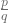(here,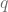can not be 0). So, we can say that any fraction with a non-zero denominator is a rational number.

What are irrational numbers?

Simply put, all real numbers that are not rational numbers are irrational numbers. i.e any real number that can not be expressed in the formNow that basic terms are out of the way, we are now ready to have recoil the summary from class 9th.

1. Let’s assume that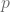andare two integers, if these two integers are written in the form, then they will be called the rational numbers which is denoted with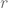. Here,can not be zero.
2. We read that the decimal expansion of a number that is rational will either be terminated or non-terminating recurring.
3. Also, if there is a number whose decimal expansion is non-terminating non-recurring, then it will be called an irrational number.
4. Considering if there is a rational number (say, it should not be equal to 0) and an irrational number (say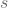), then the resulting number after adding, subtracting, multiplying, and dividing these two numbers will be an irrational number.
5. While performing different operations on two real numbers (say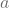and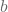) the following will be the results.
1.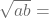2.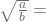3.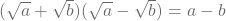4.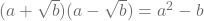5.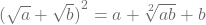6. Whenever there is a need to rationalize a denominator of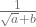, it should be multiplied by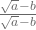. here, a and b are integers.
7. If p and q are two rational numbers then…..here a > 0.
1.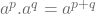2.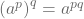3.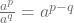4.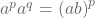### Class 10 Maths Chapter 1 Real Numbers Explanation Part 1

This summary is more than enough to brush up your memory and set the rhythm for the concept and advanced topics that we will learn in this chapter. Now let’s move on to the topics that we will learn in this class.

# Euclid’s Division Algorithm

Before we go on explaining what is Euclid’s division algorithm, let’s first understand what is an algorithm. An algorithm is a term that you will mostly hear in math and computer programming, an algorithm is a set of well-defined and well-structured instructions that are used to solve or computing problems.

One of the best real examples of an algorithm is making tea, there are a series of steps that one follows to make tea, like step 1: putting the pan on the stove, step 2: adding water, etc.

Now coming back to Euclid’s division algorithm, it is a series of steps by which we can find the HCF (highest common factor) of any two integers.

# Euclid’s Division Lemma

Again, to understand Euclid’s division lemma, we must first be familiar with the term ‘lemma’. So, when a statement that is true is used to test the truth of another statement is called a lemma. For the sake of simplicity, you can think of the lemma as logic that will be used to prove something.

Euclid’s division lemma states that if there are two positive numbers (sayand) then there exist unique integersandsuch that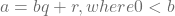.

You will practice a lot of questions based on Euclid’s division lemma in class 10 maths chapter 1 real numbers.

#### Class 10 Maths Chapter 1 Real Numbers Explanation Part 2

Besides Euclid’s division algorithm, another important concept or topic of the chapter real numbers in class 10 is the fundamental theorem of arithmetic. Using this theorem, you will need to find the LCM and HCF of given numbers in chapter 1 exercise 1.2. Let’s take a quick look at what this theorem is all about.

# Fundamental Theorem of Arithmetic

The fundamental theorem of arithmetic states that “every composite number can be expressed (factorized) as a product of primes, and this factorization is unique, apart from the order in which the prime factors occur”.

Simply put, this theorem tells that every composite number can be written as the product of the powers of prime numbers. You also know it as the prime factorization method from your previous class. Besides these theorems, there are five more theorems in the class 10th NCERT book.

# Revisiting Rational Numbers

From here on the NCERT book elaborates on the facts that you had studied in class 9th. This part especially goes on to prove how numbers under square root are irrational with 3 solved examples.

# Revisiting Rational Numbers And Their expansion

This part of the chapter explains how we can find if the decimal expansion of the rational number is terminating or non-terminating repeating (or recurring).

Let’s take a look at the blueprint of class 10 maths chapter 1 real numbers.

Maths Chapter 1 Blueprint For class 10 (Real Numbers )
1 Marker 1 question
2 Marker 1 question
3 marker 1 question
Total questions 3
Total marks 6

Before proceeding, please note that the given blueprint is based on the latest sample paper released by CBSE. The number of questions and marks assigned to them may be different in board exams. Students are advised to prepare accordingly.

As you can see in the table, the marking scheme for real numbers in class 10th is made in proportion, which means that there is something for all levels. According to the current marking scheme, a total of 3 questions will be asked from this chapter for a total of 6 marks, 1 question for 1 marker, 1 for 2 markers & 1 for 3 markers.

So, that’s it for this chapter, we will keep on adding study material and other important links here. If you have anything that you want to ask then you can write us using the comment section.